# Boys to girls

The ratio of boys to girls at a party is 3:5. If six more boys arrived and four girls left the party, the ratio of boys to girls would be 5:6. How many people were at the party initially?

t =  64

### Step-by-step explanation:Did you find an error or inaccuracy? Feel free to write us. Thank you!Tips to related online calculators
Need help to calculate sum, simplify or multiply fractions? Try our fraction calculator.
Check out our ratio calculator.
Do you have a linear equation or system of equations and looking for its solution? Or do you have a quadratic equation?

## Related math problems and questions:

• School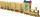School attend 792 children, boys and girls ratio is 4:5. How many more girls go to school (than boys)?
• Numbers at ratio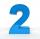The two numbers are in a ratio 3:2. If we each increase by 5 would be at a ratio of 4:3. What is the sum of original numbers?
• PupilsThere are 32 pupils in the classroom, and girls are two-thirds more than boys. a) How many percents are more girls than boys? Round the result to a whole percentage. b) How many boys are in the class? c) Find the ratio of boys and girls in the class. Writ
• Party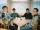At the party everyone clink with everyone. Together, they clink 406 times. How many people were at the party?There are 15 boys and 12 girls at the graduation party. Determine how many four couples can be selected.
• Boys and girls 8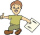In a class of 35 boys and girls that has a ratio of 2:5, 5 boys are absent and 5 boys are present. How many students are present?
• ChildrenThe group has 42 children. There are 4 more boys than girls. How many boys and girls are in the group?
• ClassroomThere are eighty more girls in the class than boys. Boys are 40 percent and girls are 60 percent. How many are boys and how many girls?
• Boys and girls 2The ratio of boys to girls in math club is 4:3 . After 8 more girls joined the Club, the ratio become 1:1. How many members are there in the club now?
• Glasses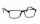There are 36 pupils in the class. Nine girls wear glasses. Boys with glasses are five less than girls without glasses. Boys without glasses are two times more than girls without glasses. How many boys and how many girls?
• Euler problem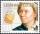Someone buys a 180 tolars towels. If it was for the same money of 3 more towels, it would be 3 tolars cheaper each. How many were towels?
• Lookout towerHow high is the lookout tower? If each step was 3 cm lower, 60 more of them were on the lookout tower. If it were 3 cm higher again, it would be 40 less than it is now.
• School parliament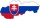There are 18 boys and 14 girls in the class. In how many ways can 3 representatives be elected to the school parliament, if these are to be: a) the boys themselves b) one boy and two girls
• Prospering studentsThere are girls and 30 boys in the class. Boys prospering 28, girls all. How many girls are in the class and how many boys are there, if all the prospering students are 95%?
• At theAt the presentation of the travelers came three times as many men than women. When eight men left with their partners, there were five times more men than women at the presentation. How many were men and women originally?
• Summer camp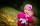84 children spent their holidays at the summer camp. There were 8 fewer boys than girls. What percentage were boys and what percentage were girls?
• The ratioThe ratio of girls to boys in Mrs. White's class is 3:2.  If there are 12 boys, how many girls are in the classroom?